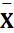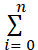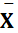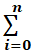or# RD Sharma Class 12 and Chapter Mean And Variance Of A Random Variable Maths Solutions

## Maths Class 12 and Chapter Mean And Variance Of A Random Variable RD Sharma SolutionsFilter
Filters :
CLASSES
•
•
•
•
•
•
•
CHAPTERS
•
• 24 More

### RD SHARMA Class 12 | MEAN AND VARIANCE OF A RANDOM VARIABLE

The random variable X has a probability distribution P(X) of the following form, where k is some number : P(X) = `{(k ,if x=0) , (2k ,if x=1), (3k ,if x=2), (0 otherwise)` (a) Determine k. (b) Find P `( X lt 2)` , P `( X le 2)` , P` (X ge 2 )`The class 12th board examination is one of the most important exams in the academic life of a student.  Marks obtained in higher secondary exam play a pivotal role in one’s career. These marks are referred to during the admission in a college or a job interview. One can easily infer the importance of class 12 from the above discussion. One cannot take these exams as a cake walk because a lot of students attempt board examinations every year, but only a few are able to score really good marks. Only with sheer determination and hard work, one can excel the board exams and ensure better academic and professional life ahead.  Smart work and time management are important for achieving success and right study material for every subject is a must have for every student to obtain excellent marks in class 12.

Many students buy a number of books for each subject due to the lack of right guidance and they don’t even study comprehensively with any one of them as the material provided in the book is not up to the mark, or they end up studying all the books that lead to confusion in every concept.  It is very important to have a right strategy to excel in an exam and the goal of a student should not be to go through a number of books, but to solve a single comprehensive reference book intensively.  Some students refer to NCERT books solutions that help to an extent but largely fails in satisfying the need for the examination. NCERT books for other subjects can prove to be useful, but for mathematics, it’s doesn’t stand a chance. As we know, mathematics is all about rigorous practice and questions provided in NCERT books are just a handful. Therefore, it is necessary for every student who wants to excel in the board examinations that they buy a reference book for maths.

RD Sharma Mathematics Book for Class 12 is the most talked book among CBSE students for maths as it has helped the students to obtain high grades in their board examinations.  It has been a bible for CBSE students to prepare mathematics for board exams. The RD Sharma book provides everything needed for a good score as the detailed and in-depth theories explained in an easy to understand language.

However, the book does not give solutions to all the questions in the books.  This issue is easily resolved by online websites that are dedicated to providing quality solutions to each and every problem for the book of RD SHARMA.

We at Doubtnut, an online learning platform, believe that every single student is entitled to quality education so we provide solutions that are easy to follow so that every single student can be benefited. The solutions provided are of top-notch quality and easily available. These solutions have been prepared by keeping in mind the latest trends and patterns in CBSE boards. Each step and method used in the solutions are given in well-structured format so that students can learn from them without any confusions.

The solutions are prepared by experts and highly experienced faculty and it is also ensured that every solution is 100% accurate. The solutions provided will not only help students in preparations of exams, but also in completing their daily homework.

Chapter 32 of RD SHARMA FOR CLASS 12 is Mean and Variance of a Random Variable. The solutions given are well-framed and every formula and methods used are well highlighted. It makes sure to not to include the formulae or methods in the solutions that have not been introduced to students until that chapter.

## Solutions for Chapter 33 Mean and Variance of a Random Variable

The chapter of mean and variance of a random variable is a concept in probability, which is introduced for the first time in class 12th. It is small and easy to understand the chapter and a good amount of questions have been asked from this chapter in previous years.

In this chapter, one will study the concept of a random variable, discrete random variable and continue random variable.

1. Random variable: Correspondence between the elements of a sample space associated with a random experiment and set of a real number is defined as a random variable.
2. Discrete random variable: If a random variable assumes a countable number of values, it is called discrete random variable.
3. Continuous random variable: If a random variable assumes uncountable number of values, it is called continuous random variable.

The solutions provided by subject matter experts at doubtnut are prepared keeping in mind that every student be it someone who is weak in maths or has a strong grip of the subject, can understand the concept and method used in solving the particular problem clearly and swiftly.  These solutions will help students develop their own algorithms for solving problems and will also advance the ability to disintegrate every problem in a logical manner. Students following these solutions will learn new tricks and alternative methods for questions. These solutions will enable students to get better at maths in every way possible.

Students can follow these solutions and find out the areas in which they need to improve and also evaluate their mistakes done in solving problems and rectify them. In this way, students will eliminate their weak concepts and build stronger ones.

The basic formula that has been used by many toppers to excel in mathematics is regular practice as it is the mantra of success in mathematics.  One should start his/her practice of maths from the book of RD SHARMA from the starting of the academic year and should devote at least 2 to 3 hours every day in solving problems from the RD Sharma book. The correct way to read or study any book is that first one should read the theory given thoroughly, make notes for later reference, and then dedicate complete two hours of practicing the solved exercise given after the explanation of the same. Students should try to solve each and every question on their own before looking at the solutions only when one is not able to solve a given problem after several tries should look at these solutions. Students can refer to these solutions to rectify their mistakes. Learning this way will definitely ensure high marks in mathematics board examinations.

## Topics and Subtopics in Chapter 32 Mean And Variance Of A Random Variable

The book of R.D. SHARMA is remarkably well structured and it is the reason that makes this book so comprehensive. The complete book is divided into two volumes and the text follows the latest syllabus and trends in CBSE board examinations.

Each chapter is divided into small and easy to understand topics and subtopics that reduces the complexity of concepts. The theory is provided for these topics and subtopics, which is very comprehensive and detailed and is followed by problems on the same. The problems provided in the book are in variety to help students to understand patterns of questions asked in board exams. The questions are arranged in ascending order of their difficulty level. At the end of the topic, unsolved problems are also provided for revision by students.

The text is illustrated beautifully with necessary graphs, diagrams, charts and real-life examples which deepens the understanding of a student on the given topic.

Some of the topics and subtopics in which chapter 33 is divided are as follows:

### Discrete Random Variable

Let s be the sample space associated with a random variable experiment. Then, a real-valued function X which assigns to each event w € S to a unique real number X(w) is called a random variable.

### Probability Distribution

If a random variable X takes values x1, x2, x3, ……., xn with respect to probabilities p1, p2, p3, ……., pn, then

X :            x1              x2               ………                xn

P( X ) :           p1              p2               ……..                pn

is known as the probability distribution of X.

### Mean of A Discrete Random Variable

If X is a discrete random variable which assumes values x1, x2, x3, ……., xn with respective probabilities p1, p2, p3, ……., pn, then the mean X-of X is defined as

X-= p1 x1 + p2 x2 + ….. + pn  xn

Or, X-=  n i= 0pi  xi

1. POINT 1: The mean of random variable X is also known as its mathematical expectation or expected value and is denoted by E ( X ).
2. POINT 2: In case of a frequency distribution xi/ fi ; i = 1, 2, ……., n the mean X-Is given by

X-=   1 ( f1 x1 + f2 x2 + ……. + fn xn )

N

### Variance of A Random Discrete Variable

If X is discrete random variable which assumes values x1, x2, x3, ……., xn with the respective probabilities p1, p2, p3, ……., pn, then the variance of X is defined as

Var(X) = p1 ( x1- X -)2  + p2 ( x2- X -)2 + …….. + pn( xn - X -)2

Or,         Var ( X ) = n i=0pi (xi - X-)2

## Significance of RD Sharma mathematics book for class 12

Some of the highlighting features of the book are as follows:

• The RD Sharma book provides a detailed and comprehensive theory on every topic that helps students to excel the subject in a better way.
• In the book, previous year and NCERT EXEMPLAR problems are given for every topic.
• Short notes and formulae are also provided at the end of each topic for a quick revision.
• MCQs provided at the end of the chapter for students preparing for competitive exams.